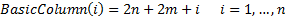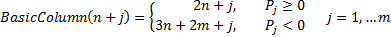/

### Constrained Quadratic Optimisation: 3. Setting up the ‘super’-problem

Next page

3.            Setting up the ‘super’-problem

To set up the superset of the original problem as described in Solving the canonical problem, we multiply through each row in the above tableau (both left and right hand sides) by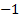if the relevant element of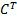or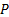is negative. This means that the right-hand side of the tableau is all now non-negative. Simultaneously we introduce a further series of ‘artificial’ variables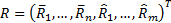, all elements of which are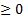, the firstelements of which,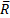, are artificial variables corresponding to the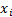and the remaining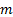elements are artificial variables corresponding to the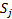. To be more precise, if we want the speediest algorithm, we only introduce only such elements of these variables that are needed to achieve a starting feasible solution.

The starting solution is then given by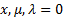and: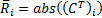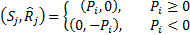The starting ‘basic’ variables, i.e. those whose opening values are greater than zero are each of the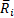and whichever of theand the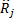is non-zero in the above formulae.

The complete starting tableau is then: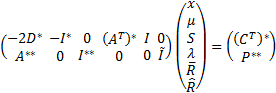Subject to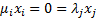for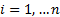,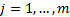and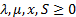where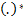means multiply through relevant row of the matrix byif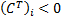,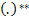means multiply through relevant row of the matrix byif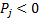andis the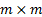identity matrix with each 1 replaced by a zero if the corresponding value of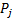is greater than zero.

N.B. We have ignored for the purposes of this discussion the possibility that any of the entries on the right-hand side of the tableau are identically zero. This degenerate case can be handled by adding a very small number to make them positive.

We need to know which variables ‘correspond’ to each other (i.e. appear jointly in the constraints, although these correspondences do not change as the iteration progresses.

In addition to the Tableau, which is an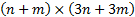array we also need to keep track of the following as the iteration proceeds:

Two rows:

(a)    BasicRow, indicates with, say, a 1 whether the variable in question is ‘basic’

(b)   ObjectiveRow, initially calculated as: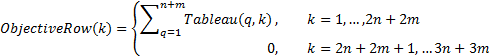Two columns:

(c)    SolutionColumn, contains the current feasible solution, i.e. the right hand side of the above tableau ‘equation’; and

(d)   BasicColumn, contains integers indicating to which variables the entries in the SolutionColumn currently apply (and thus which variables are basic, so there is some overlap here with BasicRow). BasicColumn starts as: